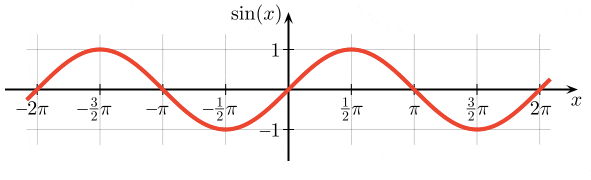Constructive Interference

Homework Statement
A radio transmitting station operating at a frequency f=100 MHz has two identical antennas that radiate in phase (in other words, coherent with respect to each other). Antenna B is 10 m to the right of antenna A. Consider the point P between the antennas, and along the line connecting them a horizontal distance x to the right of antenna A.

a) What is the wavelength of the electromagnetic wave?
b) At point P, what is the path difference (PD) between the waves coming from the two antennas (give your answer in terms of x)?
c) For what values of x will constructive interference occur at point P?
d) How many constructive interference points will be there on the line between the two antennas?

Relevant Equations
c=λf
v=λf?

The attempt at a solution
a.) c=λf, so λ=(3*108m/s)/(1*108Hz)=3m. This gave me the correct answer according to the study guide, but I've seen some people use v (speed of sound) instead of c (speed of light). I'm not sure which is correct.
b.) PD= (10-x)-x= 10-2x
c.) Constructive interference occurs when PD= mλ. So, x=-(mλ)/20. For m values 1-4, x= -0.15, 0.3, 0.45, 0.6 According to the answer key this is wrong, but I don't understand how to get the right answer.
d.) The total distance is 10m, so I would keep plugging in m values until I get an x ≥10.

Merlin3189
Homework Helper
Gold Member
...
a.) c=λf, so λ=(3*108m/s)/(1*108Hz)=3m. This gave me the correct answer according to the study guide, but I've seen some people use v (speed of sound) instead of c (speed of light). I'm not sure which is correct. Do radio waves travel at the speed of light or at the speed of sound?
b.) PD= (10-x)-x= 10-2x Yes
c.) Constructive interference occurs when PD= mλ. So, x=-(mλ)/20. For m values 1-4, x= -0.15, 0.3, 0.45, 0.6 According to the answer key this is wrong, but I don't understand how to get the right answer. If PD=mλ. and PD=10 -2x then mλ.=10 -2x which does not rearrange to your expression
d.) The total distance is 10m, so I would keep plugging in m values until I get an x ≥10.

•not_a_physics_major
berkeman
Mentor
Homework Statement
A radio transmitting station operating at a frequency f=100 MHz has two identical antennas that radiate in phase (in other words, coherent with respect to each other). Antenna B is 10 m to the right of antenna A. Consider the point P between the antennas, and along the line connecting them a horizontal distance x to the right of antenna A.

a) What is the wavelength of the electromagnetic wave?
b) At point P, what is the path difference (PD) between the waves coming from the two antennas (give your answer in terms of x)?
c) For what values of x will constructive interference occur at point P?
d) How many constructive interference points will be there on the line between the two antennas?

Relevant Equations
c=λf
v=λf?

The attempt at a solution
a.) c=λf, so λ=(3*108m/s)/(1*108Hz)=3m. This gave me the correct answer according to the study guide, but I've seen some people use v (speed of sound) instead of c (speed of light). I'm not sure which is correct.
b.) PD= (10-x)-x= 10-2x
c.) Constructive interference occurs when PD= mλ. So, x=-(mλ)/20. For m values 1-4, x= -0.15, 0.3, 0.45, 0.6 According to the answer key this is wrong, but I don't understand how to get the right answer.
d.) The total distance is 10m, so I would keep plugging in m values until I get an x ≥10.
Welcome to the PF.For EM waves, use the velocity c. For sound waves, use the speed of sound for the velocity. Makes sense?

And you get constructive interference when the path length difference is a multiple of 2π, not just π. You can see that by looking at the plot of a sinusoidal waveform. See how the wave repeats itself every 2π? If you add two waves that are π apart in phase, they cancel each other out. Can you see that now?ADD -- Sorry, I mistread your post. You did say when the PD is a multiple of the wavelength, not of π.EDIT -- Merlin types faster than I do!Attachments

Chandra Prayaga
b.) PD= (10-x)-x= 10-2x
c.) Constructive interference occurs when PD= mλ. So, x=-(mλ)/20.
The calculation for x went wrong. If you start from mλ = 10 - 2x, you don't get x = -(mλ)/20.

The calculation for x went wrong. If you start from mλ = 10 - 2x, you don't get x = -(mλ)/20.
Thank you! I suspected there was an algebra error somewhere!

•berkeman
Welcome to the PF.For EM waves, use the velocity c. For sound waves, use the speed of sound for the velocity. Makes sense?

And you get constructive interference when the path length difference is a multiple of 2π, not just π. You can see that by looking at the plot of a sinusoidal waveform. See how the wave repeats itself every 2π? If you add two waves that are π apart in phase, they cancel each other out. Can you see that now?ADD -- Sorry, I mistread your post. You did say when the PD is a multiple of the wavelength, not of π.

EDIT -- Merlin types faster than I do!•